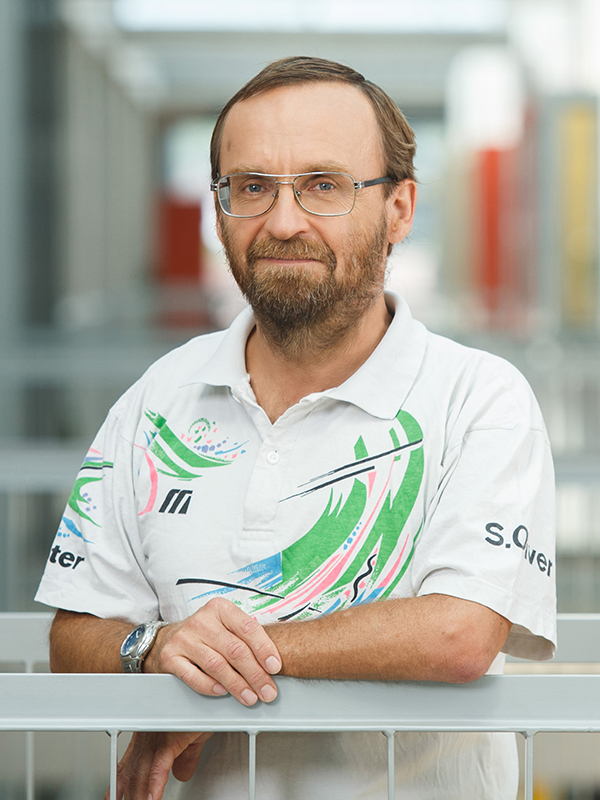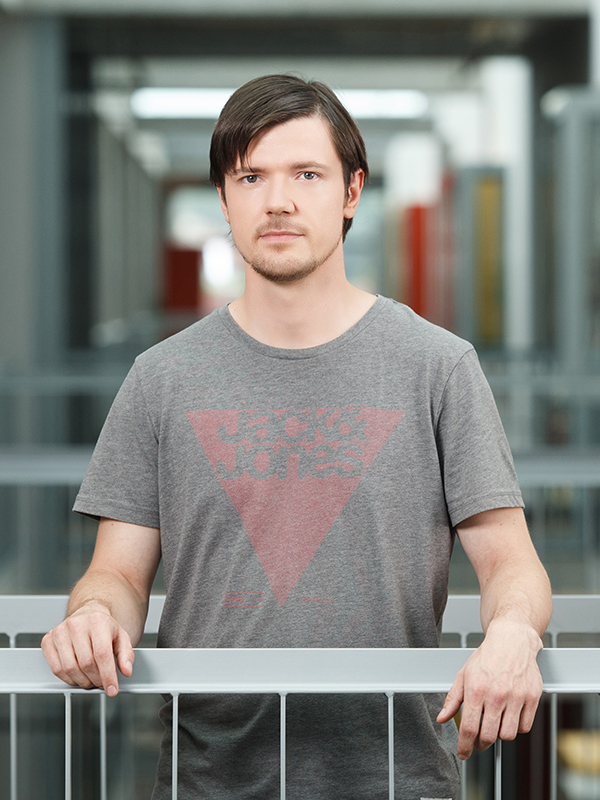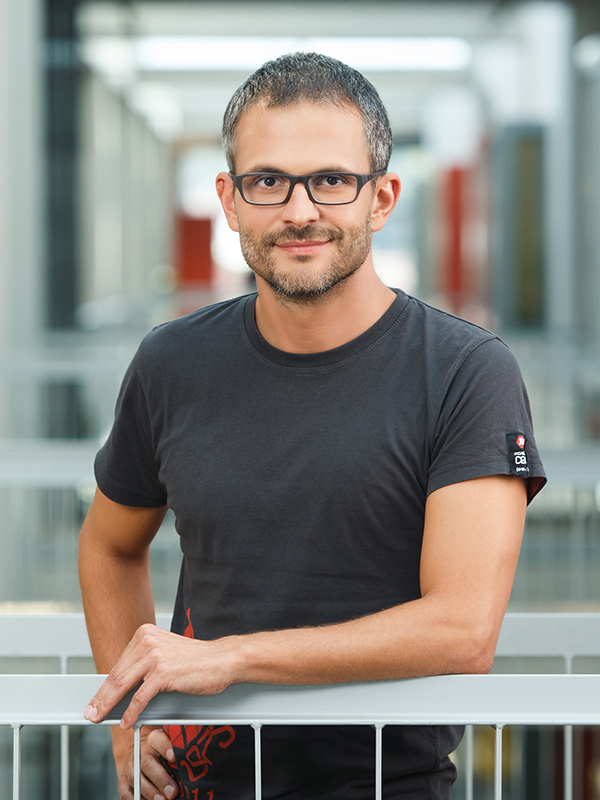• Course code:63519
• Credits:6
• Semester: winter
• Contents

Machine learning is used in industry, medicine, economy etc. for data analysis and knowledge discovery from databases, data mining, for generating knowledge bases for expert systems, for learning predictions and recognitions, playing games, understanding natural language, hand-writing, speech, images etc. A basic principle of machine learning is decribing (modelling) of phenomena from data. The result of learning are rules, functions, relations, equations, and probabilistic distributions. The trained models try to explain data and can be used for decision making during the observation of the modelled process in future. The goal of the course is to present the teoretical basics and basic priciples of machine learning methods, basic machine learning algorithms and their usage in practice for knowledge discovery from data and for learning classification and regression models. Students will apply the theoretical knowledge on real world problems from science and economy.

Overview of course contents:  What is learning and relation between learning and intelligence, ML basics, Advanced attribute evaluation measures, Advanced methods for estimating performance of ML, Advanced visualization methods, Combining ML algorithms, Bayesian learning, Calibration of probabilities, Explanation of individual predictions, Numerical ML methods, Artificial neural networks: RBF, Deep NN, Unsupervised learning: clustering, Association rules, Estimating the reliability of individual predictions, Text mining, Matrix factorization, Arcehtypal analysis, ML as data compression, active learning, user porfiling and recommendation systems, ILP, Introduction to learning theory.

Practical part is in the form of solving problems and web quizzes and completing the seminar work. Assistant is available for consultations. The grade of practical work is the grade of the seminar work. The precondition for passing practical work is achieving at least 50% of points in web quizzes.

The final course grade consists of practical work grade (50%) and exam (50%). On the written exam students need to achieve at least 50% of points.

• Study programmes
• Distribution of hours per semester
45
hours
lectures
24
hours
laboratory work
6
hours
tutorials
• ProfessorInstructor
Room:R2.07 - KabinetTeaching Assistant
Room:R2.26 - Laboratorij LKMTeaching Assistant
Room:R2.26 - Laboratorij LKM